## Spring Design

Design information for engineers, such as spring calculation formulas,

which are the basis of spring design, can be found here.

### Meaning of Symbols

The symbols used for spring design are shown in Table 1 below. The values of the longitudinal elastic modulus (E) are based on Table 2.

Table 1. Symbols and units used in calculations

Symbol Meaning of Symbol Unit
Ac Cross-sectional area of the outer ring
Ai
rc Center of gravity radius of outer ring cross-section
ri Radius of center of gravity of the inner ring cross-section
a Angle of contact surface
ρ Friction angle
δ Spring deflection mm
c Spring index　c=D/d
n Number of contact surfaces
R1 Spring constant when pressurizing
R2 Spring constant when decompressing
E Longitudinal elastic modulus N/mm2

Table 2.Longitudinal elastic modulus：E（N/m㎡）

Material E Value
Spring steel material 206×103
Stainless steel

SUS302

SUS304

SUS631

186×103
186×103
196×103

### Spring Constant and Load

The basic formula for the spring constant and load at the time of pressurization are calculated with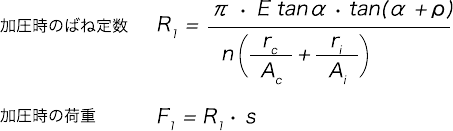The basic formula for the spring constant and load during decompression can be calculated as follows.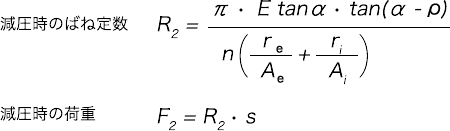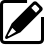Product information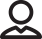Our Proposals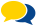Popular questions